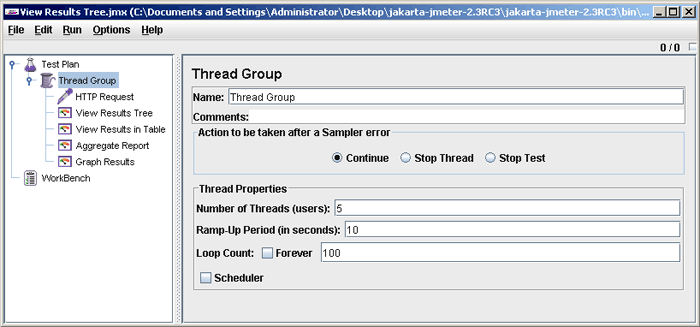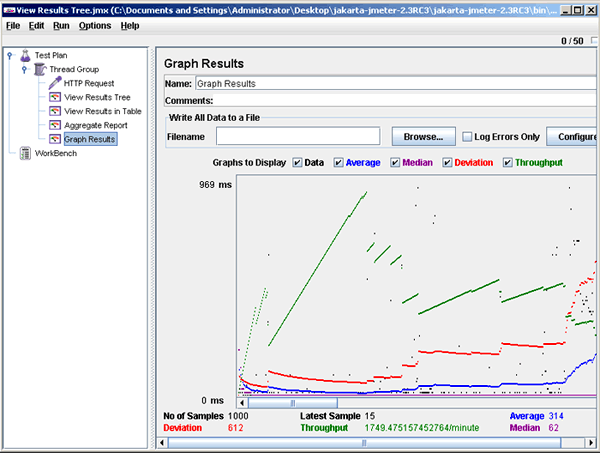Tutorials

You can also view the graph for your test after running it. For this go to Thread group -> Add -> Listener -> Graph results as shown in the picture below. After clicking on the graph element, you will see the chart getting populated with the data represen

You can also view the graph for your test after running it. For this go to Thread group -> Add -> Listener -> Graph results as shown in the picture below. After clicking on the graph element, you will see the chart getting populated with the data represen

You can also view the graph for your test after running it. For this go to Thread group -> Add -> Listener -> Graph results as shown in the picture below. After clicking on the graph element, you will see the chart getting populated with the data representing requests to the server.The chart  above shows the Test results with number of threads = 5, ramp-up time = 10, loop count = 100, and loop controller set to 1.

Three parameters on the charts have been displayed - throughput (green line), median (purple line) and average (blue line). If we modify the parameters to simulate a higher load on a server by increasing the number of threads to 50, and changing the ramp-up time to 5 and loop count to 20, then we get the following graph by clicking the start again.The meaning of the parameters at the bottom of the chart is as follows:

• The number of requests processed by the server per minute is denoted by Throughput.
• Average is being calculated as the total time running divided by number of requests sent to the server.
• Time is represented by Median i.e. server's half response time is lower than this number.
• The variation in the server's response time is denoted by Deviation.
• The completion of any last request is shown by Latest.

The summary of the above two runs is shown below in the form of a table.

 Number of threads Throughput, responses/minute Average, ms Median, ms 5 1665 57 31 50 1749 314 62

You can also add the Spline Visualizer to further visualize the test results of all sample times. It provides a smooth view of distribution based on polynomial approximations as a continuous line. The picture below displays the screen of Spline Visualizer.The same test results have been shown using Spline Visualizer.